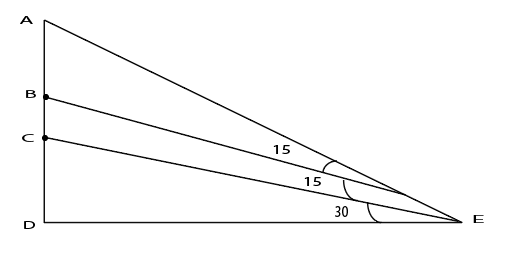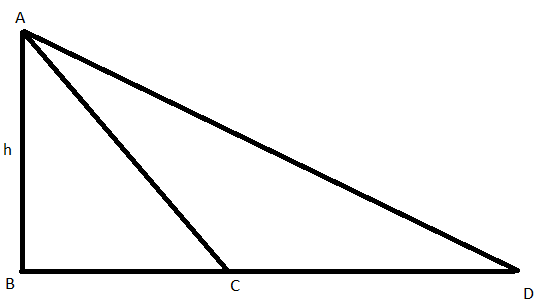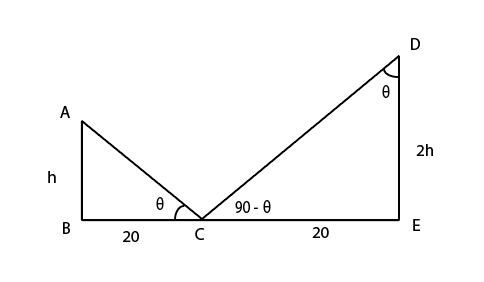0
557

# Angle Of Elevation & Depression Questions for XAT 2022 – Download PDF

Download Angle of Elevation and Depression Questions for XAT PDF – XAT Angle of Elevation and Depression questions pdf by Cracku. Top 10 very Important Angle of Elevation and Depression Questions for XAT based on asked questions in previous exam papers.

Question 1: The topmost point of a perfectly vertical pole is marked A. The pole stands on a flat ground at point D. The points B and C are somewhere between A and D on the pole. From a point E, located on the ground at a certain distance from D, the points A, B and C are at angles of 60, 45 and 30 degrees respectively. What is AB : BC : CD?

a) $(3 + \sqrt 3) : (1 + \sqrt 3) : 1$

b) $(3 – \sqrt 3) : 1 : (\sqrt 3 – 1)$

c) 1 : 1 : 1

d) $(3 – \sqrt 3) : (\sqrt 3 – 1) : 1$

e) $(\sqrt 3 – 1) : 1 : (3 – \sqrt 3)$

Question 2: A man standing on the line joining the two poles finds that the top of the poles make an angle of elevation of $60^\circ$ and $45^\circ$ respectively. After walking for sometime towards the other pole, the angles change to $30^\circ$ and $60^\circ$ respectively. The ratio of the height of the poles is :

a) $\frac{\sqrt{3}-1}{2}$

b) $\frac{\sqrt{3}+1}{3}$

c) $\frac{\sqrt{3}-1}{4}$

d) $\frac{\sqrt{3}+1}{4}$

Question 3: Peter was standing on the top of a rock cliff facing the sea. He saw a boat coming towards the shore. As he kept seeing time just flew. Ten minutes less than half of an hour, the angle of depression changed from 30 to 60. How much more time in minutes will the boat take to
reach the shore ?

a) 5

b) 10

c) 15

d) 20

Question 4: A flag pole on the top of a mall building is 75 m high. The height of the mall building is 325 m. To an observer at a height of 400 m, the mall building and the pole subtend equal angle $\theta$. If the horizontal distance of the observer from the pole is ‘x’, then what is the value of x?

a) $20\sqrt{10}$ m

b) $30\sqrt{10}$ m

c) $25\sqrt{5}$ m

d) None

Question 5: Triangle ABC is a right angled triangle. D and E are mid points of AB and BC respectively. Read
the following statements.
I. AE = 19
II. CD = 22
III. Angle B is a right angle.
Which of the following statements would be sufficient to determine the length of AC?

a) Statement I and Statement II.

b) Statement I and Statement III.

c) Statement II and III.

d) Statement III alone.

e) All three statements.

Question 6: Two vertical poles are 40 metres apart and the height of one is double that of the other. From the middle point of the line joining their feet, an observer finds the angular elevations of their tops to be complementary. Find their heights.

a) 14.14 m, 28.28 m

b) 12 m, 22 m

c) 12.12 m, 24.24 m

d) None of these.

Question 7: A man standing on the line joining the two poles finds that the top of the poles make an angle of elevation of $60^\circ$ and $45^\circ$ respectively. After walking for sometime towards the other pole, the angles change to $30^\circ$ and $60^\circ$ respectively. The ratio of the height of the poles is :

a) $\frac{\sqrt{3}-1}{2}$

b) $\frac{\sqrt{3}+1}{3}$

c) $\frac{\sqrt{3}-1}{4}$

d) $\frac{\sqrt{3}+1}{4}$

Question 8: A rectangular swimming pool is 48 m long and 20 m wide. The shallow edge of the pool is 1 m deep.
For every 2.6 m that one walks up the inclined base of the swimming pool, one gains an elevation of 1 m. What is the volume of water (in cubic meters), in the swimming pool? Assume that the pool is filled up to the brim.v

a) 528

b) 960

c) 6790

d) 10560

e) 12960

Question 9: Peter was standing on the top of a rock cliff facing the sea. He saw a boat coming towards the shore. As he kept seeing time just flew. Ten minutes less than half of an hour, the angle of depression changed from 30 to 60. How much more time in minutes will the boat take to
reach the shore ?

a) 5

b) 10

c) 15

d) 20

Question 10: There are two buildings, one on each bank of a river, opposite to each other. From the top of one building – 60 m high, the angles of depression of the top and the foot of the other building are 30° and 60° respectively. What is the height of the other building?

a) 30 m

b) 18 m

c) 40 m

d) 20 mLet ED = $\sqrt{\ 3}x$
In triangle CDE, $\tan\ 30\ =\ \frac{CD}{\sqrt{\ 3}x}$ => CD = x

In triangle BDE, $\tan\ 45\ =\ \frac{BD}{\sqrt{\ 3}x}$ => BD = $\sqrt{\ 3}x$ => BC = $\sqrt{\ 3}x\ -x$

In triangle ADE, $\tan\ 60\ =\ \frac{AD}{\sqrt{\ 3}x}$ => AD = 3x => AB = $3x\ -\ \sqrt{\ 3}x$

AB : BC : CD = $(3 – \sqrt 3) : (\sqrt 3 – 1) : 1$

Let ‘a’ and ‘b’ be the heights of the two poles

X be the initial position of the man and the angles of elevation be $60^0$ and $45^0$

Distance between pole 1 and X  = $\ \frac{\ a}{\sqrt{\ 3}}$ and distance between pole 2 and X = b

Let Y be the position of the man after walking sometime towards the other pole =

Distance between Y and pole 1 = $\ \ a\sqrt{\ 3}$ and distance between the pole 2 and Y = $\ \frac{\ b}{\sqrt{\ 3}}$

Since the distance between the poles will remain the same

$\ \frac{\ a}{\sqrt{\ 3}}$ + b = $\ \ a\sqrt{\ 3}$ + $\ \frac{\ b}{\sqrt{\ 3}}$

$\ \frac{\ a}{b}=\ \ \frac{\ \sqrt{\ 3}-1}{2}$

Let AB represent the rock cliff with its height be hD represents the initial point and C is the point at which he observers the boat after Ten minutes less than half of an hour (20 minutes).

BD = $\frac{h}{\tan\ \left(30\right)}\ =\ \sqrt{\ 3}h$

BC = $\frac{h}{\tan\ \left(60\right)}\ =\ \frac{h}{\sqrt{\ 3}}$

In 20 minutes boat covered CD length which is = $\sqrt{\ 3}h\ -\ \frac{h}{\sqrt{\ 3}}=\ \frac{2h}{\sqrt{\ 3}}$

Hence remaining lenght $\frac{h}{\sqrt{\ 3}}$(BC) will be covered in 10 minutes

In the given diagram AE is the mall building and DE is pole. The observer is at C point which is 400 mts from the ground.

It is given that $\angle$DCE = $\angle$ECA = $\theta$

In $\triangle$ACD and $\triangle$ECD

tan(2$\theta$) = $\frac{400}{x}$

tan($\theta$) = $\frac{75}{x}$

We know that tan(2$\theta$) = $\frac{2tan(\theta)}{1-tan^{2}(\theta)}$

$\therefore$ $\frac{400}{x}$ = $\frac{2*\frac{75}{x}}{1-(\frac{75}{x})^{2}}$

$x^{2}$ = 9000 = $30\sqrt{10}$

ABC is right angled triangle. D and E are mid points of AB and BC respectively.

As it is not given that which angle is 90°. So we need statement (III) to find the value of AC.

Whereas using statement (I) & (II) alone, we cannot find the value of AC.

But using all the three statements. We can find value of $(AB)^2 + (BC)^2$.

Hence, the value of $AC = \sqrt{(AB)^2 + (BC)^2}$ using Pythagoras Theorem.

=> Ans – (E) : All statements are required.

Let AB and CD be the vertical poles such that the height of one is double to that of the otherIt is given that the angles of elevation are complimentary. Thus let us assume the angle made by smaller tower be $\theta\$. Then angle made by DE is $90-\theta\$

In $\bigtriangleup\$ ABC,  $\tan\left(\theta\ \right) = \frac{\ h}{20}$

In $\bigtriangleup\$ CDE, $\tan\left(\theta\ \right) = \frac{\ 20}{2h}$

Equating the values of$\tan\left(\theta\right)$ we get, $\ \frac{\ h}{20}=\ \frac{\ 20}{2h}$

$h^2\ =\ 200$ $\Rightarrow$ $h=14.14$

Hence height of poles are $14.14$ and $28.28$

Let ‘a’ and ‘b’ be the heights of the two poles

X be the initial position of the man and the angles of elevation be $60^0$ and $45^0$

Distance between pole 1 and X  = $\ \frac{\ a}{\sqrt{\ 3}}$ and distance between pole 2 and X = b

Let Y be the position of the man after walking sometime towards the other pole =

Distance between Y and pole 1 = $\ \ a\sqrt{\ 3}$ and distance between the pole 2 and Y = $\ \frac{\ b}{\sqrt{\ 3}}$

Since the distance between the poles will remain the same

$\ \frac{\ a}{\sqrt{\ 3}}$ + b = $\ \ a\sqrt{\ 3}$ + $\ \frac{\ b}{\sqrt{\ 3}}$

$\ \frac{\ a}{b}=\ \ \frac{\ \sqrt{\ 3}-1}{2}$

For every 2.6 m that one walks along the slanting part of the pool, there is a height of 1 m that is gained.

=> $\frac{AC}{BC} = \frac{2.6}{1}$

=> $AC = 2.6 \times BC$

Also, dimensions of cuboidal part = $48 \times 20 \times 1$

In $\triangle$ ABC

=> $(AC)^2 = (AB)^2 + (BC)^2$

=> $(2.6 \times BC)^2 = (48)^2 + (BC)^2$

=> $6.76 (BC)^2 – (BC)^2 = 2304$

=> $(BC)^2 = \frac{2304}{5.76} = 400$

=> $BC = \sqrt{400} = 20$ m

$\therefore$ Volume of water in the pool = Volume of cuboid + Volume of triangle

= $(l \times b \times h) + (\frac{1}{2} \times AB \times BC) \times height$

= $(48 \times 20 \times 1) + (\frac{1}{2} \times 48 \times 20 \times 20)$

= $960 + 9600 = 10560 m^3$

Let AB represent the rock cliff with its height be hD represents the initial point and C is the point at which he observers the boat after Ten minutes less than half of an hour (20 minutes).

BD = $\frac{h}{\tan\ \left(30\right)}\ =\ \sqrt{\ 3}h$

BC = $\frac{h}{\tan\ \left(60\right)}\ =\ \frac{h}{\sqrt{\ 3}}$

In 20 minutes boat covered CD length which is = $\sqrt{\ 3}h\ -\ \frac{h}{\sqrt{\ 3}}=\ \frac{2h}{\sqrt{\ 3}}$

Hence remaining lenght $\frac{h}{\sqrt{\ 3}}$(BC) will be covered in 10 minutes